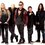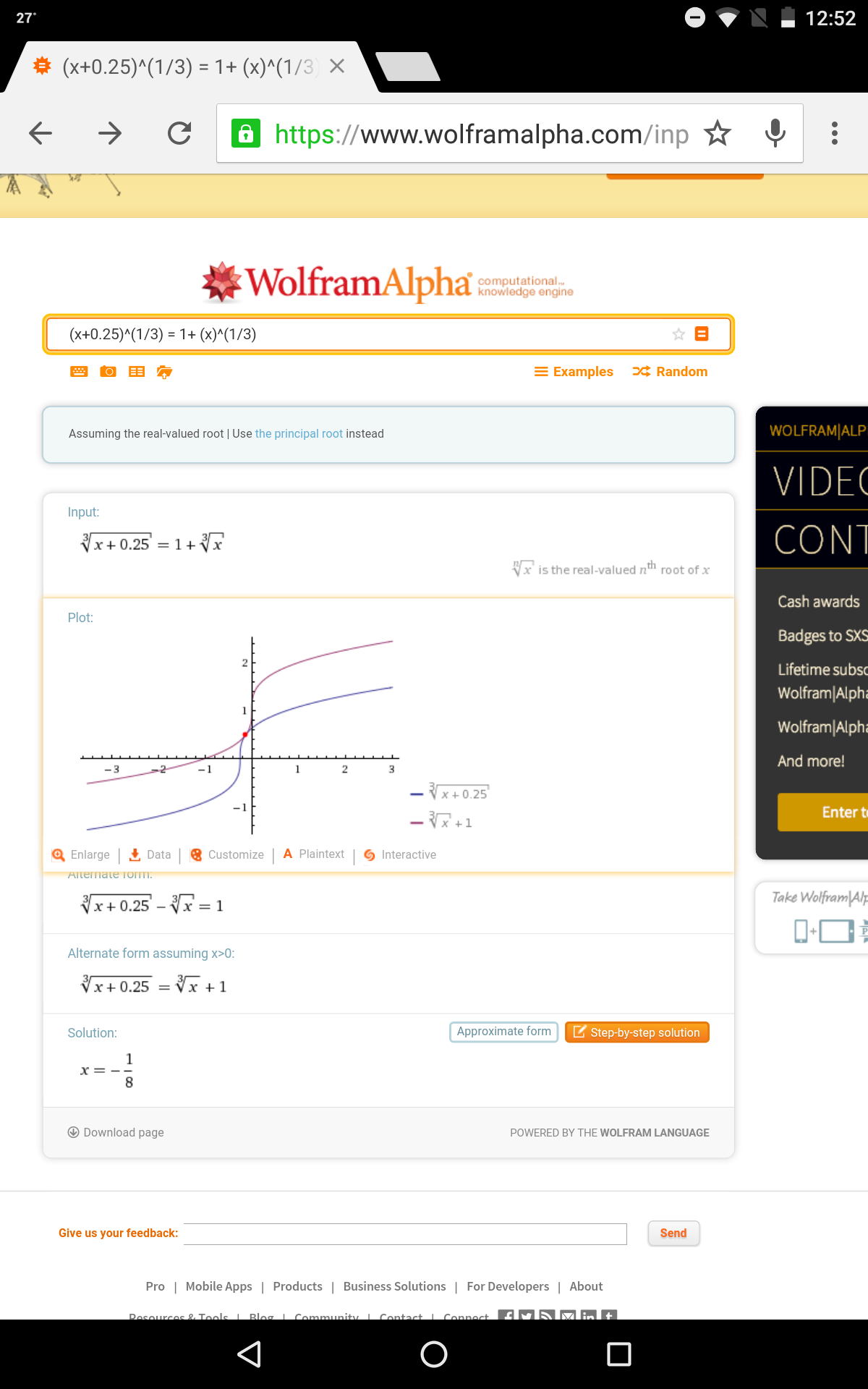# Where is the mistake?

Given that $p$ is a real number , then for what values of $p$ the equation ${(x+3p+1)}^{\frac{1}{3}} - x^ \frac{1}{3} = 1$ has real solutions.

${(x+3p+1)}^{\frac{1}{3}} - x^ \frac{1}{3} - 1 = 0$ if $a+b+c = 0$ we have $a^3+b^3+c^3 = 3abc$ $x+ 3p + 1 -x -1 = 3[(x+3p+1)(x)]^\frac{1}{3}$ $3p = 3[(x+3p+1)(x)]^\frac{1}{3}$ $p^3 = x(x + 3p +1)$ $x^2 + (3p + 1)x -p^3 =0$ For real roots $D \geq 0$ $4p^3 + 9p^2 + 6p +1 \geq 0$ $(p+1)^2(4p+1) \geq 0$ therefore , $p \geq \frac{-1}{4} \cup -1.$

Please help me understand which step did I do wrong? And what is the correct solution for the above question?Note by Ujjwal Mani Tripathi
5 years ago

This discussion board is a place to discuss our Daily Challenges and the math and science related to those challenges. Explanations are more than just a solution — they should explain the steps and thinking strategies that you used to obtain the solution. Comments should further the discussion of math and science.

When posting on Brilliant:

• Use the emojis to react to an explanation, whether you're congratulating a job well done , or just really confused .
• Ask specific questions about the challenge or the steps in somebody's explanation. Well-posed questions can add a lot to the discussion, but posting "I don't understand!" doesn't help anyone.
• Try to contribute something new to the discussion, whether it is an extension, generalization or other idea related to the challenge.

MarkdownAppears as
*italics* or _italics_ italics
**bold** or __bold__ bold
- bulleted- list
• bulleted
• list
1. numbered2. list
1. numbered
2. list
Note: you must add a full line of space before and after lists for them to show up correctly
paragraph 1paragraph 2

paragraph 1

paragraph 2

[example link](https://brilliant.org)example link
> This is a quote
This is a quote
    # I indented these lines
# 4 spaces, and now they show
# up as a code block.

print "hello world"
# I indented these lines
# 4 spaces, and now they show
# up as a code block.

print "hello world"
MathAppears as
Remember to wrap math in $$ ... $$ or $ ... $ to ensure proper formatting.
2 \times 3 $2 \times 3$
2^{34} $2^{34}$
a_{i-1} $a_{i-1}$
\frac{2}{3} $\frac{2}{3}$
\sqrt{2} $\sqrt{2}$
\sum_{i=1}^3 $\sum_{i=1}^3$
\sin \theta $\sin \theta$
\boxed{123} $\boxed{123}$

Sort by:

Now, note that

$a+b+c=0 \implies a^3 + b^3 + c^3 = 3abc$

But, $a^3 + b^3 + c^3 = 3abc \not \Rightarrow a+b+c=0$

So, $a+b+c=0$ is not equivalent to $a^3 + b^3 + c^3 = 3abc$.

A better approach to solve this is putting $x=y^3 \iff x^{\frac{1}{3}} = y$.

Then, the equation becomes:

\begin{aligned}(y^3 + 3p +1)^{\frac{1}{3}} &= 1+y \\\iff y^3 + 3p +1 &= (1+y)^3 \\\iff y^2 + y -p &= 0 \end{aligned}

So, for the roots of the original equation to be real, we need the discriminant of $y^2 + y - p=0$ to be non negative.

That is, $1+4p \geq 0 \\\iff p \geq -\frac{1}{4}$

I got what you meant here but still i think the answer is wrong , check on wolframalpha putting p = -0.25 , it says no solution exists to the below equation for ${(x+0.25)}^{\frac{1}{3}} - x^ \frac{1}{3} - 1 = 0$

- 5 years ago

Try $x = \frac{-1}{8}$Oh yes , i was working only with principal root value and hence was getting no solution

- 5 years ago

WolframAlpha gives you the option to "use the real-valued root instead", (in blue just below the entry box), which in this case will give you a value of $x = -\frac{1}{8}$ as Deeparaj has pointed out.

- 5 years ago

What does $\frac{-1}{4} \cup 1$ mean?

i mean that p>= -0.25 and also p = -1 is a solutionof the inequality
$(p+1)^2(4p+1) \geq 0$

- 5 years ago

@Brian Charlesworth @Jon Haussmann , your help will be valuable .

- 5 years ago

I used the same approach as Deeparaj, but I think we need more than just the discriminant to be non-negative; in order to avoid complex values for $y = x^{\frac{1}{3}}$ we will require that $x \ge 0$ and thus also $y \ge 0$. So we require that

$y = \dfrac{-1 + \sqrt{1 + 4p}}{2} \ge 0 \Longrightarrow \sqrt{1 + 4p} \ge 1 \Longrightarrow p \ge 0$,

which seems to be in agreement with results from WolframAlpha.

- 5 years ago

Don't we define $x^{\frac{1}{3}} = -(-x)^{\frac{1}{3}} \quad x < 0$ right?

Yes, we could choose to work with the real-valued root, in which case WolframAlpha does return a value of $x = -\frac{1}{8}$ for $p = -\frac{1}{4}$, and no solutions if $p \lt -\frac{1}{4}$.

(WolframAlpha can be a bit difficult when taking fractional roots of negative numbers.)

- 5 years ago

this is exactly what i was confused about . Thank you for helping me out with the wolframalpha query .

- 5 years ago